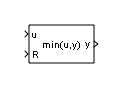# MinMax Running Resettable

Determine minimum or maximum of signal over time

• Library:

•## Description

The MinMax Running Resettable block outputs the minimum or maximum of all past inputs `u`. You specify whether the block outputs the running minimum or maximum with the Function parameter.

The block can reset its state based on an external reset signal `R`. When the reset signal `R` is nonzero (`true`), the block resets the output to the value of the Initial condition parameter.

The input can be a scalar, vector, or matrix signal. The block outputs a signal having the same dimensions as the input. Each output element equals the running minimum or maximum of the corresponding input elements.

## Ports

### Input

expand all

Input signal as a scalar, vector, or matrix. Based on what you specify for the Function parameter, the block outputs the minimum or maximum value of all past inputs `u`.

If you specify a scalar value for the Initial condition parameter, the block expands the parameter to have the same dimensions as nonscalar input `u`.

Data Types: `single` | `double` | `int8` | `int16` | `int32` | `int64` | `uint8` | `uint16` | `uint32` | `uint64` | `Boolean` | `fixed point` | `bus`

The input port accepting the reset signal as a scalar, vector, or matrix. When the reset signal is nonscalar, it must have the same dimensions as input signal `u`. As long as the reset signal has a value of zero, the block outputs the running minimum or maximum value of input `u`. Whenever the reset signal has a nonzero value (`true`), the block resets the output to the value of the Initial condition parameter.

Data Types: `single` | `double` | `int8` | `int16` | `int32` | `int64` | `uint8` | `uint16` | `uint32` | `uint64` | `Boolean` | `fixed point` | `bus`

### Output

expand all

Output signal, specified as a scalar, vector, or matrix, where each output element equals the running minimum or maximum value of the corresponding input elements. Output signal `y` has the same data type and dimensions as input signal `u`.

When the block receives a nonzero (`true`) reset signal, the block resets the output to the value of the Initial condition parameter.

Data Types: `single` | `double` | `int8` | `int16` | `int32` | `int64` | `uint8` | `uint16` | `uint32` | `uint64` | `Boolean` | `fixed point`

## Parameters

expand all

Specify whether the block outputs the running minimum or maximum value of the corresponding input elements.

#### Programmatic Use

 Block Parameter: `Function` Type: character vector Values: `'min' | 'max'` Default: `'min'`

Specify the initial condition value. When the reset input signal `R` is `true`, the block resets the output to the value you specify.

#### Programmatic Use

 Block Parameter: `vinit` Type: character vector Values: scalar or vector Default: `'0.0'`

## Block Characteristics

 Data Types `Boolean` | `double` | `fixed point` | `integer` | `single` Direct Feedthrough `no` Multidimensional Signals `no` Variable-Size Signals `no` Zero-Crossing Detection `no`

## Version History

Introduced before R2006a